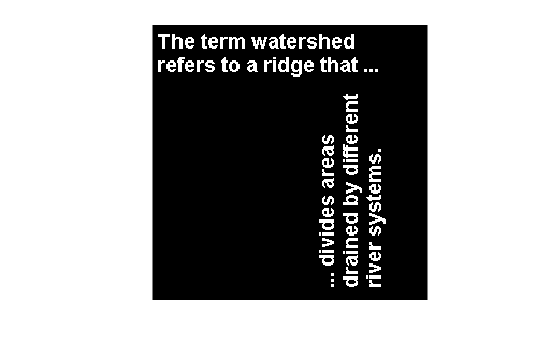Documentation

# labelmatrix

Create label matrix from `bwconncomp` structure

## Syntax

``L = labelmatrix(CC)``

## Description

example

````L = labelmatrix(CC)` creates a label matrix, `L`, from the connected components structure `CC` returned by `bwconncomp`.`labelmatrix` is more memory efficient than `bwlabel` and `bwlabeln` because it returns its label matrix in the smallest numeric class necessary for the number of objects.```

## Examples

collapse all

Read a binary image into the workspace.

`BW = imread('text.png');`

Calculate the connected components using `bwconncomp`.

`CC = bwconncomp(BW);`

Create a label matrix using `labelmatrix`.

`L = labelmatrix(CC);`

For comparison, create a second label matrix using `bwlabel`.

`L2 = bwlabel(BW);`

View both label matrices in the workspace. Note that `labelmatrix` uses the smallest numeric class necessary for the number of objects and therefore is more memory efficient than `bwlabel` .

`whos L L2`
``` Name Size Bytes Class Attributes L 256x256 65536 uint8 L2 256x256 524288 double ```

Display the label matrix as an RGB image using `label2rgb`. To make it easier to differentiate the different connected components, shuffle the color order of the labels.

`imshow(label2rgb(L,'jet','w','shuffle'));`## Input Arguments

collapse all

Connected components, specified as a structure with four fields.

FieldDescription
`Connectivity`Connectivity of the connected components (objects)
`ImageSize`Size of the binary image
`NumObjects`Number of connected components (objects) in the binary image.
`PixelIdxList`1-by-`NumObjects` cell array where the k-th element in the cell array is a vector containing the linear indices of the pixels in the k-th object.

## Output Arguments

collapse all

Label matrix of contiguous regions, returned as matrix of nonnegative integers. The pixels labeled `0` are the background. The pixels labeled `1` make up one object; the pixels labeled `2` make up a second object; and so on.

The size of `L` is `CC.ImageSize`. The class of `L` depends on `CC.NumObjects`, as shown in the table.

ClassRange
`'uint8'`

`CC.NumObjects` $\le$ 255

`'uint16'`

256 $\le$ `CC.NumObjects` $\le$ 65535

`'uint32'`

65536 $\le$ `CC.NumObjects` $\le {2}^{32}-1$

`'double'`

`CC.NumObjects` $\ge {2}^{32}$

Data Types: `double` | `uint8` | `uint16` | `uint32`